# Mode

Go back to  'Data'

## Introduction

When describing a set of data, the central position of the data set is identified. This is known as the central measure of tendency. The three most common measures of central tendency are mean, median and mode.

Know more about the measures of central tendency.

Learn about the Mean and the Median.

## Mode

In any collection of numbers, the number which occurs the most number of times is the mode.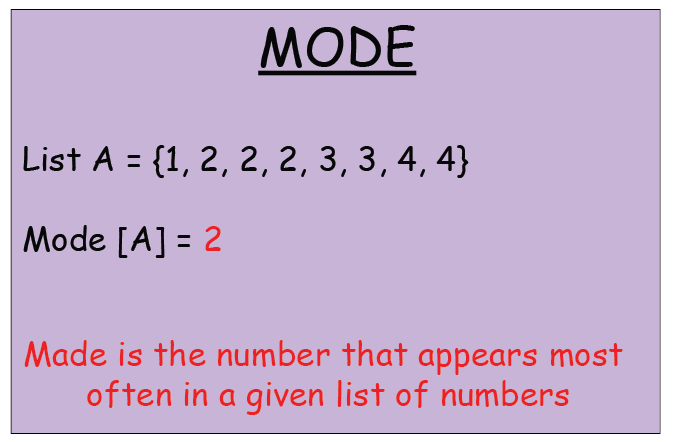A list can be unimodal, bimodal, trimodal or multimodal.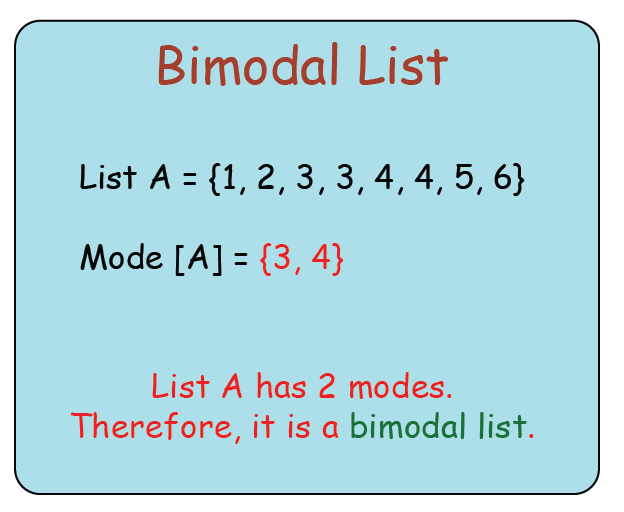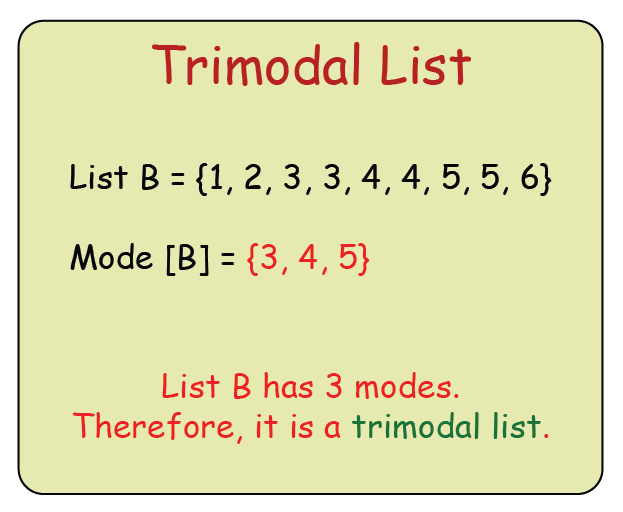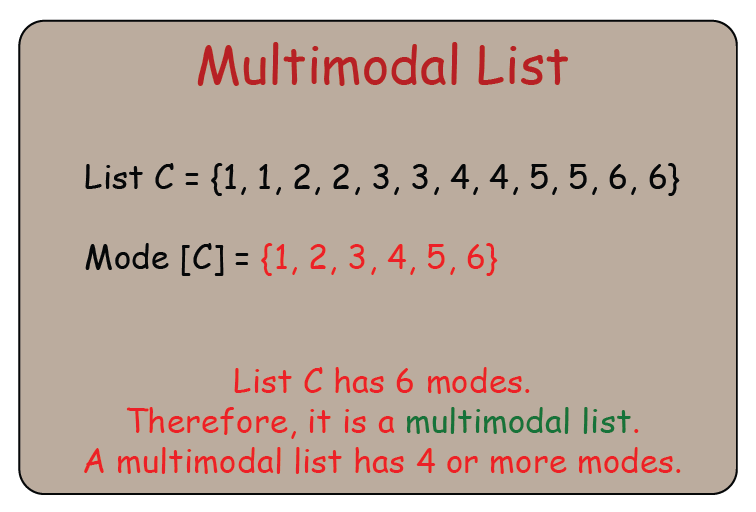When we need to calculate the mode in the case of grouped frequency distribution, we will first identify the modal class, the class that has the highest frequency. Then, we will use the formula given below to calculate the mode.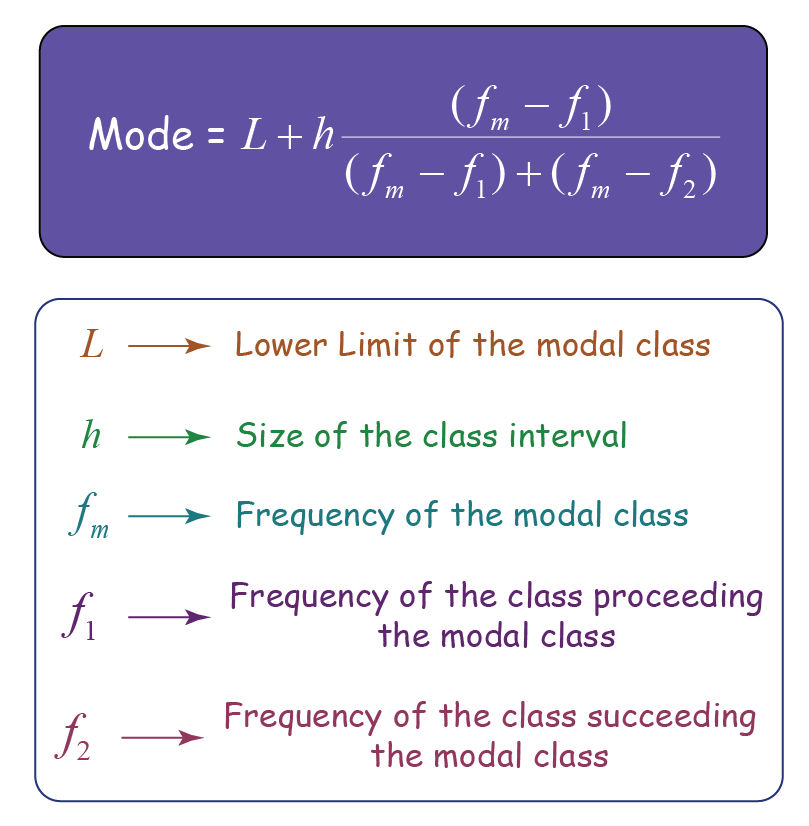Let’s consider the following table.

 Marks obtained in Science Number of students $$100\text{-}90$$ $$5$$ $$90\text{-}80$$ $$10$$ $$80\text{-}70$$ $$2$$ $$70\text{-}60$$ $$12$$ $$60\text{-}50$$ $$1$$

Modal class is $$70\text{-}80$$
\begin{align}L&=70\\h&=10\\f_m&=12\\f_1&=2\\f_2&=1\end{align}

Substituting the values in the formula, we will get

\begin{align} \text{Mode}&= 70 + 10 * \left[ \frac{(12-2)}{(12-2)+ (12-1)} \right] \\ &= 70 + 10 * \left[ \frac{10}{(10+11)} \right] \\ &= 70 + 10 * \left[ \frac{10}{21} \right] \\ &= 70 + 4.76\\ &= 74.76 \\ \end{align}

$\boxed{\;\;\;\text{Mode}= 74.76\;\;\;}$

## Solved Examples

### Example 1

Find the mode for the given data set.

{$$3, 3, 3, 4, 4, 4, 4, 5, 5, 6$$}

The mode for the above given set is {$$4$$}

### Example 2

Find the mode for the given data set.

{$$23, 30, 32, 34, 45, 45, 54, 54, 59, 68$$}

The modes for the above given set are {$$45, 54$$}

### Example 3

The table given below lists the height (in centimeters) of $$30$$ students in Grade 9. Calculate the mode for the given dataset.

 Height (in centimetres) Number of students $$150\text{-}145$$ $$5$$ $$145\text{-}140$$ $$7$$ $$140\text{-}135$$ $$6$$ $$135\text{-}130$$ $$9$$ $$130\text{-}125$$ $$3$$

This is an example of grouped frequency distribution.

We will first identify the modal class.

The highest frequency is $$9.$$

Thus, the modal class is $$135\text{-}130$$

Now, let us apply the formula given below to calculate the mode.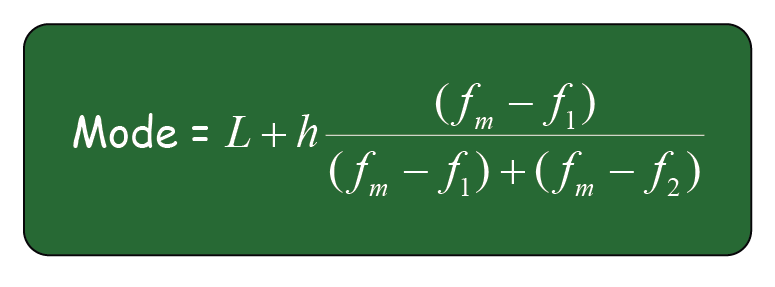\begin{align}L&=135\\h&=5\\f_m&=9\\f_1&=6\\f_2&=3 \end{align}

Substituting the values in the formula, we will get

\begin{align}\text{Mode} &= 135 + 5 * \left[\frac{( 9 - 6 )} {(9 - 6) + (9-3)}\right]\\ &= 135 + 5 * \left[ \frac{3} {(3 + 6)} \right]\\ &= 135 + 5 * \left[ \frac{3}{9} \right]\\ &= 135 + 1.67\\ &= 136.67 \end{align}

$\boxed{\;\;\;\text{Mode}=136.67\;\;\;}$

## Practice Questions

### Question 1

Find the mode of the data set given below.

{$$1, 2, 2, 3, 4, 5, 6, 7, 8$$}

Hint: The data item that appears the most number of times is the Mode.

### Question 2

Find the mode of the data set given below.

{$$11, 22, 23, 24, 25, 26, 27, 28$$}

Hint: It is possible for a data set to have NO Mode.

### Question 3

The table given below lists the quantity of rice donated by different housing societies. Calculate the mode for the given dataset.

 Quantity of rice (in kgs) Number of housing societies $$1\text{-}5$$ $$7$$ $$5\text{-}10$$ $$10$$ $$10\text{-}15$$ $$9$$ $$15\text{-}20$$ $$4$$

Hint: This is an example of grouped frequency distribution. Use the formula given below.

More Important Topics
Numbers
Algebra
Geometry
Measurement
Money
Data
Trigonometry
Calculus
More Important Topics
Numbers
Algebra
Geometry
Measurement
Money
Data
Trigonometry
Calculus
Learn from the best math teachers and top your exams

• Live one on one classroom and doubt clearing
• Practice worksheets in and after class for conceptual clarity
• Personalized curriculum to keep up with school

0